Open in App
Not now

# Association, Composition and Aggregation in Java

• Difficulty Level : Medium
• Last Updated : 18 Oct, 2022

Association is a relation between two separate classes which establishes through their Objects. Association can be one-to-one, one-to-many, many-to-one, many-to-many. In Object-Oriented programming, an Object communicates to another object to use functionality and services provided by that object. Composition and Aggregation are the two forms of association.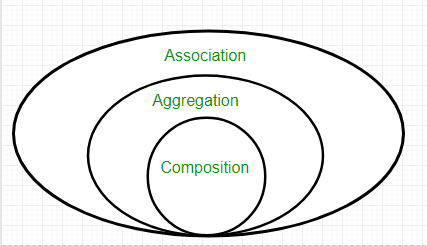Example:

## Java

 `// Java Program to illustrate the` `// Concept of Association`   `// Importing required classes` `import` `java.io.*;`   `// Class 1` `// Bank class` `class` `Bank {`   `    ``// Attributes of bank` `    ``private` `String name;` `    `  `      ``private` `Set employees;` `    ``// Constructor of this class` `    ``Bank(String name)` `    ``{` `        ``// this keyword refers to current instance itself` `        ``this``.name = name;` `    ``}`   `    ``// Method of Bank class` `    ``public` `String getBankName()` `    ``{` `        ``// Returning name of bank` `        ``return` `this``.name;` `    ``}` `  `  `      ``public` `setEmployees(Set employees){` `        ``this``.employees = employees;` `    ``}` `  ``public` `getEmployees(Set employees){` `        ``return` `this``.employees;` `    ``}` `}`   `// Class 2` `// Employee class` `class` `Employee {` `    ``// Attributes of employee` `    ``private` `String name;` `    ``// Employee name` `    ``Employee(String name)` `    ``{` `        ``// This keyword refers to current instance itself` `        ``this``.name = name;` `    ``}`   `    ``// Method of Employee class` `    ``public` `String getEmployeeName()` `    ``{` `        ``// returning the name of employee` `        ``return` `this``.name;` `    ``}` `}`   `// Class 3` `// Association between both the` `// classes in main method` `class` `GFG {`   `    ``// Main driver method` `    ``public` `static` `void` `main(String[] args)` `    ``{`   `        ``// Creating objects of bank and Employee class` `        ``Bank bank = ``new` `Bank(``"ICICI"``);` `        ``Employee emp = ``new` `Employee(``"Ridhi"``);`   `        ``Set employees = ``new` `HashSet<>();` `          ``employees.add(emp);` `      `  `          ``bank.setEmployees(employees);` `      `  `        ``System.out.println(bank.getEmployees()+``"are belongs to bank"``+bank.getBankName());` `    ``}` `}`

Output

`Ridhi is employee of ICICI`

Output Explanation: In the above example, two separate classes Bank and Employee are associated through their Objects. Bank can have many employees, So it is a one-to-many relationship.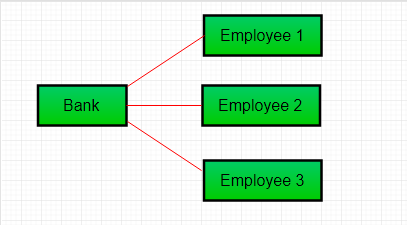### Aggregation

It is a special form of Association where:

• It represents Has-A’s relationship.
• It is a unidirectional association i.e. a one-way relationship. For example, a department can have students but vice versa is not possible and thus unidirectional in nature.
• In Aggregation, both the entries can survive individually which means ending one entity will not affect the other entity.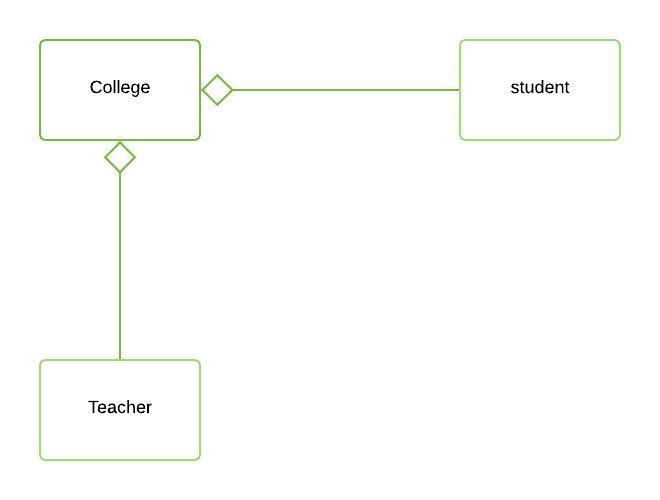Aggregation

Example

## Java

 `// Java program to illustrate Concept of Aggregation`   `// Importing required classes` `import` `java.io.*;` `import` `java.util.*;`   `// Class 1` `// Student class` `class` `Student {`   `    ``// Attributes of student` `    ``String name;` `    ``int` `id;` `    ``String dept;`   `    ``// Constructor of student class` `    ``Student(String name, ``int` `id, String dept)` `    ``{`   `        ``// This keyword refers to current instance itself` `        ``this``.name = name;` `        ``this``.id = id;` `        ``this``.dept = dept;` `    ``}` `}`   `// Class 2` `// Department class contains list of student objects` `// It is associated with student class through its Objects` `class` `Department {` `    ``// Attributes of Department class` `    ``String name;` `    ``private` `List students;` `    ``Department(String name, List students)` `    ``{` `        ``// this keyword refers to current instance itself` `        ``this``.name = name;` `        ``this``.students = students;` `    ``}`   `    ``// Method of Department class` `    ``public` `List getStudents()` `    ``{` `        ``// Returning list of user defined type` `        ``// Student type` `        ``return` `students;` `    ``}` `}`   `// Class 3` `//  Institute class contains list of Department` `// Objects. It is associated with Department` `// class through its Objects` `class` `Institute {`   `    ``// Attributes of Institute` `    ``String instituteName;` `    ``private` `List departments;`   `    ``// Constructor of institute class` `    ``Institute(String instituteName,List departments)` `    ``{` `        ``// This keyword refers to current instance itself` `        ``this``.instituteName = instituteName;` `        ``this``.departments = departments;` `    ``}`   `    ``// Method of Institute class` `    ``// Counting total students of all departments` `    ``// in a given institute` `    ``public` `int` `getTotalStudentsInInstitute()` `    ``{` `        ``int` `noOfStudents = ``0``;` `        ``List students;`   `        ``for` `(Department dept : departments) {` `            ``students = dept.getStudents();`   `            ``for` `(Student s : students) {` `                ``noOfStudents++;` `            ``}` `        ``}`   `        ``return` `noOfStudents;` `    ``}` `}`   `// Class 4` `// main class` `class` `GFG {`   `    ``// main driver method` `    ``public` `static` `void` `main(String[] args)` `    ``{` `        ``// Creating object of Student class inside main()` `        ``Student s1 = ``new` `Student(``"Mia"``, ``1``, ``"CSE"``);` `        ``Student s2 = ``new` `Student(``"Priya"``, ``2``, ``"CSE"``);` `        ``Student s3 = ``new` `Student(``"John"``, ``1``, ``"EE"``);` `        ``Student s4 = ``new` `Student(``"Rahul"``, ``2``, ``"EE"``);`   `        ``// Creating a List of CSE Students` `        ``List cse_students = ``new` `ArrayList();`   `        ``// Adding CSE students` `        ``cse_students.add(s1);` `        ``cse_students.add(s2);`   `        ``// Creating a List of EE Students` `        ``List ee_students` `            ``= ``new` `ArrayList();`   `        ``// Adding EE students` `        ``ee_students.add(s3);` `        ``ee_students.add(s4);`   `        ``// Creating objects of EE and CSE class inside` `        ``// main()` `        ``Department CSE = ``new` `Department(``"CSE"``, cse_students);` `        ``Department EE = ``new` `Department(``"EE"``, ee_students);`   `        ``List departments = ``new` `ArrayList();` `        ``departments.add(CSE);` `        ``departments.add(EE);`   `        ``// Lastly creating an instance of Institute` `        ``Institute institute = ``new` `Institute(``"BITS"``, departments);`   `        ``// Display message for better readability` `        ``System.out.print(``"Total students in institute: "``);`   `        ``// Calling method to get total number of students` `        ``// in institute and printing on console` `        ``System.out.print(institute.getTotalStudentsInInstitute());` `    ``}` `}`

Output

`Total students in institute: 4`

Output Explanation: In this example, there is an Institute which has no. of departments like CSE, EE. Every department has no. of students. So, we make an Institute class that has a reference to Object or no. of Objects (i.e. List of Objects) of the Department class. That means Institute class is associated with Department class through its Object(s). And Department class has also a reference to Object or Objects (i.e. List of Objects) of the Student class means it is associated with the Student class through its Object(s).

It represents a Has-A relationship. In the above example: Student Has-A name. Student Has-A ID. Student Has-A Dept. Department Has-A Students as depicted from the below media.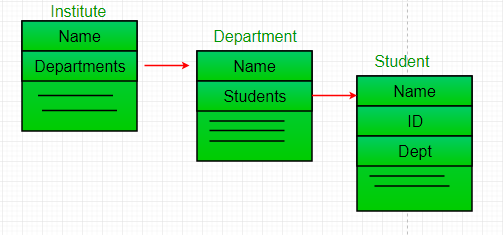When do we use Aggregation ??
Code reuse is best achieved by aggregation.

Concept 3: Composition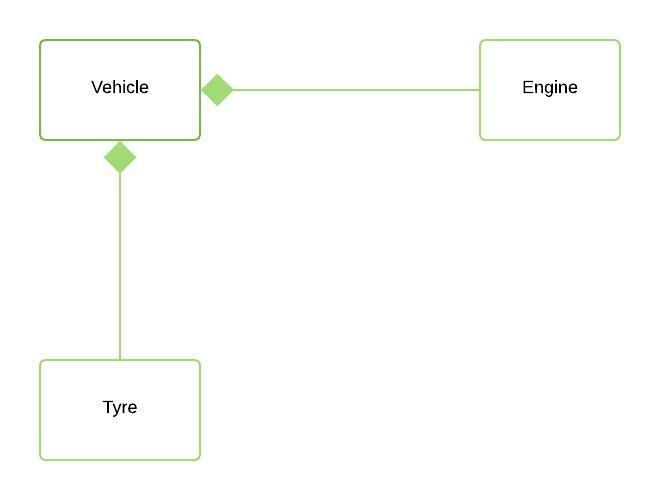Composition

Composition is a restricted form of Aggregation in which two entities are highly dependent on each other.

• It represents part-of relationship.
• In composition, both entities are dependent on each other.
• When there is a composition between two entities, the composed object cannot exist without the other entity.

Example Library

## Java

 `// Java program to illustrate` `// the concept of Composition`   `// Importing required classes` `import` `java.io.*;` `import` `java.util.*;`   `// Class 1` `//  Book` `class` `Book {`   `    ``// Attributes of book` `    ``public` `String title;` `    ``public` `String author;`   `    ``// Constructor of Book class` `    ``Book(String title, String author)` `    ``{`   `        ``// This keyword refers to current instance itself` `        ``this``.title = title;` `        ``this``.author = author;` `    ``}` `}`   `// Class 2` `class` `Library {`   `    ``// Reference to refer to list of books` `    ``private` `final` `List books;`   `    ``// Library class contains list of books` `    ``Library(List books)` `    ``{`   `        ``// Referring to same book as` `        ``// this keyword refers to same instance itself` `        ``this``.books = books;` `    ``}`   `    ``// Method` `    ``// To get total number of books in library` `    ``public` `List getTotalBooksInLibrary()` `    ``{`   `        ``return` `books;` `    ``}` `}`   `// Class 3` `// Main class` `class` `GFG {`   `    ``// Main driver method` `    ``public` `static` `void` `main(String[] args)` `    ``{`   `        ``// Creating objects of Book class inside main()` `        ``// method Custom inputs` `        ``Book b1` `            ``= ``new` `Book(``"EffectiveJ Java"``, ``"Joshua Bloch"``);` `        ``Book b2` `            ``= ``new` `Book(``"Thinking in Java"``, ``"Bruce Eckel"``);` `        ``Book b3 = ``new` `Book(``"Java: The Complete Reference"``,` `                           ``"Herbert Schildt"``);`   `        ``// Creating the list which contains number of books` `        ``List books = ``new` `ArrayList();`   `        ``// Adding books` `        ``// using add() method` `        ``books.add(b1);` `        ``books.add(b2);` `        ``books.add(b3);`   `        ``Library library = ``new` `Library(books);`   `        ``// Calling method to get total books in library` `        ``// and storing it in list of user0defined type -` `        ``// Books` `        ``List bks = library.getTotalBooksInLibrary();`   `        ``// Iterating over books using for each loop` `        ``for` `(Book bk : bks) {`   `            ``// Printing the title and author name of book on` `            ``// console` `            ``System.out.println(``"Title : "` `+ bk.title` `                               ``+ ``" and "` `                               ``+ ``" Author : "` `+ bk.author);` `        ``}` `    ``}` `}`

Output

```Title : EffectiveJ Java and  Author : Joshua Bloch
Title : Thinking in Java and  Author : Bruce Eckel
Title : Java: The Complete Reference and  Author : Herbert Schildt```

Output explanation: In the above example, a library can have no. of books on the same or different subjects. So, If Library gets destroyed then All books within that particular library will be destroyed. i.e. books can not exist without libraries. That’s why it is composition.  Book is Part-of Library.

## Aggregation vs Composition

1. Dependency: Aggregation implies a relationship where the child can exist independently of the parent. For example, Bank and Employee, delete the Bank and the Employee still exist. whereas Composition implies a relationship where the child cannot exist independent of the parent. Example: Human and heart, heart don’t exist separate to a Human

2. Type of Relationship: Aggregation relation is “has-a” and composition is “part-of” relation.

3. Type of association: Composition is a strong Association whereas Aggregation is a weak Association.

Example:

## Java

 `// Java Program to Illustrate Difference between` `// Aggregation and Composition`   `// Importing I/O classes` `import` `java.io.*;`   `// Class 1` `// Engine class which will` `// be used by car. so 'Car'` `// class will have a field` `// of Engine type.` `class` `Engine {`   `    ``// Method to starting an engine` `    ``public` `void` `work()` `    ``{`   `        ``// Print statement whenever this method is called` `        ``System.out.println(` `            ``"Engine of car has been started "``);` `    ``}` `}`   `// Class 2` `// Engine class` `final` `class` `Car {`   `    ``// For a car to move,` `    ``// it needs to have an engine.`   `    ``// Composition` `    ``private` `final` `Engine engine;`   `    ``// Note: Uncommented part refers to Aggregation` `    ``// private Engine engine;`   `    ``// Constructor of this class` `    ``Car(Engine engine)` `    ``{`   `        ``// This keywords refers to same instance` `        ``this``.engine = engine;` `    ``}`   `    ``// Method` `    ``// Car start moving by starting engine` `    ``public` `void` `move()` `    ``{`   `        ``// if(engine != null)` `        ``{` `            ``// Calling method for working of engine` `            ``engine.work();`   `            ``// Print statement` `            ``System.out.println(``"Car is moving "``);` `        ``}` `    ``}` `}`   `// Class 3` `// Main class` `class` `GFG {`   `    ``// Main driver method` `    ``public` `static` `void` `main(String[] args)` `    ``{`   `        ``// Making an engine by creating` `        ``// an instance of Engine class.` `        ``Engine engine = ``new` `Engine();`   `        ``// Making a car with engine so we are` `        ``// passing a engine instance as an argument` `        ``// while creating instance of Car` `        ``Car car = ``new` `Car(engine);`   `        ``// Making car to move by calling` `        ``// move() method inside main()` `        ``car.move();` `    ``}` `}`

Output

```Engine of car has been started
Car is moving ```

In case of aggregation, the Car also performs its functions through an Engine. but the Engine is not always an internal part of the Car. An engine can be swapped out or even can be removed from the car. That’s why we make The Engine type field non-final.